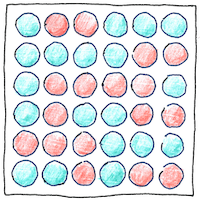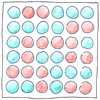# 2003 Fermionic condensate

## The book of science

Tom Sharp

 Deborah Jin, Rudolf Grimm, Wolfgang Ketterle physics## Fermionic condensate

• Superfluidic
• supercool
• fermionic
• condensates
• avoid their
• mutual
• avoidance
• by forming
• bosonic pairs.

## Holes in the theory

• If you cool them in separate magnetic domains
• until they are cold enough to combine as pairs,
• fermions can move fast without losing energy,
• but they form quantized vortices when they slow down,
• creating holes in the material until it loses
• its superfluidity.

## States of matter

• Solid, liquid, gas.
• It used to be simple,
• like A, B, C.
• We didn’t know plasmas
• danced before our eyes,
• or liquid crystals would dazzle,
• or protic organic ionic plastic crystals
• would conduct protons in fuel cells.
• Scientists tempt strange states of matter
• at the extremes of pressure and temperature
• to tease us with the hypothesis
• that the world we live in is just as strange
• and potentially transient.

According to theory, fermions have half-integer spins (an odd number of halves), unlike bosons, which have integer spins. Leptons such as electrons are fermions; quarks are fermions. The rules for adding odd numbers are analogous; two odds make an even; an odd number of odds make an odd. Composites of odd numbers of leptons and quarks are fermions. Protons and neutrons are composed of three quarks, so they are fermions. A helium-3 atom, with two protons, one neutron, and two electrons, is a fermion.